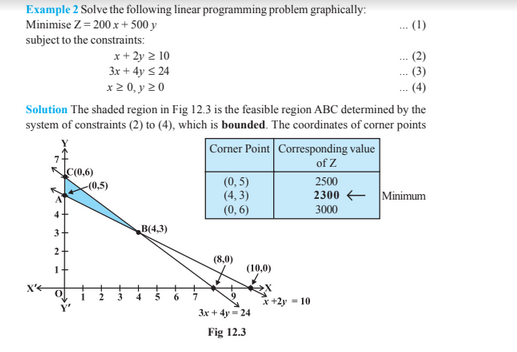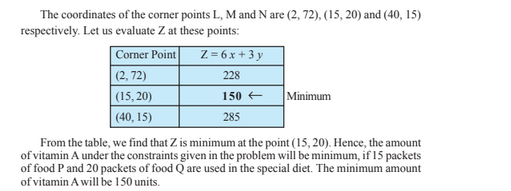# Linear Programming For Class 12

Linear programming Class 12 maths concepts help to find the maximization or minimization of the various quantities from a general class of problem. This kind of problem is known as an optimization problem. The linear programming for class 12 concepts includes finding a maximum profit, minimum cost or minimum use of resources, etc. These notes are provided here for the ease of the students to learn during the exams.

In this article, let us understand the optimization technique for a problem with the help of an example.

## Linear Programming Class 12 Concepts

In class 12, linear programming concepts covered are:

• Introduction
• Linear programming problems and mathematical formulation
• A mathematical formulation of the problems
• Graphical method of solving linear programming problems
• Different types of linear programming problems

Different Type of Linear Programming Problems

The various types of problem in linear programming problem included in class 12 concepts. They are:

(i) Manufacturing problem- Here we maximize the profit with the help of minimum utilization of the resource.

(ii) Diet Problem- We determine the number of different nutrients in a diet to minimize the cost of manufacturing.

(iii) Transportation problem- Here we determine the schedule to find the cheapest way of transporting a product at minimum time.

### Linear Programming Class 12 Sample ProblemLet us take one of the examples of diet problem.For more information on class 12 Maths concepts, register with BYJU’S – The Learning App and also explore more Maths-related videos.